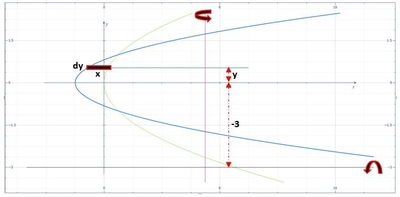# Consider the region bounded by the x-axis and the curves x = 3y^2 - 2 and x = y^2. Express, as an...

## Question:

Consider the region bounded by the x-axis and the curves {eq}x = 3y^2 - 2 {/eq} and {eq}x = y^2 {/eq}. Express, as an integral, the volume of the region obtained by rotating this region about:

a. the line x = 7.

b. the line y = -3.

Insert context explanation here...

Below is the graph,The intersections of the two curves are,

{eq}\displaystyle 3y^{2}-2=y^{2} {/eq}

{eq}\displaystyle 2y^{2}=2 {/eq}

{eq}y=-1,1 {/eq}

a.) From the graph,

{eq}\displaystyle r_{1}=7-\left ( 3y^{2} -2\right ),\:r_{2}=7-y^{2},\:h=dy {/eq}

Thus,

{eq}\displaystyle V=\pi \int_{-1}^{1}\left [ \left ( 7-\left ( 3y^{2} -2\right )\right )^{2}-\left ( 7-y^{2}\right )^{2} \right ]dy {/eq}

{eq}\displaystyle V=\pi \int_{-1}^{1}\left ( 8y^4-40y^2+32 \right )dy {/eq}

Integrate,

{eq}\displaystyle V=\pi \left [ \frac{8y^5}{5}-\frac{40y^3}{3}+32y \right ]^{1}_{-1} {/eq}

{eq}\displaystyle V=\frac{608\pi }{15} {/eq}

b.) From the graph,

{eq}\displaystyle r=y-(-3)=y+3,\:h=x,\:dr=dy {/eq}

Thus,

{eq}\displaystyle V=2\pi \int_{-1}^{1}\left ( y+3 \right )xdy {/eq}

{eq}\displaystyle V=2\pi \int_{-1}^{1}\left ( y+3 \right )\left [ y^{2}-\left ( 3y^{2}-2 \right ) \right ]dy {/eq}

{eq}\displaystyle V=2\pi \int_{-1}^{1}\left ( -2y^3-6y^2+2y+6 \right )dy {/eq}

Integrate,

{eq}\displaystyle V=2\pi \left [ -\frac{y^4}{2}-2y^3+y^2+6y \right ]^{1}_{-1} {/eq}

{eq}\displaystyle V=16\pi {/eq}